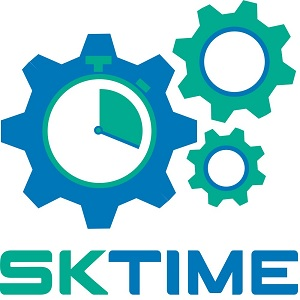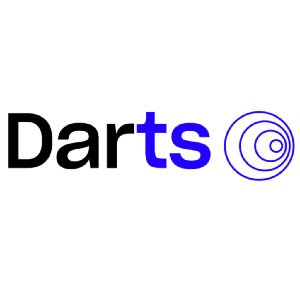Machine Learning for Timeseries

A brief summary of the topics covered in this course is as below. This is 24 hours course, it is suggested to complete this course in 3 weeks. Apart from my classroom course, you will be given exercises, and it will take another 100 hours in the course duration to complete these exercises.

## Introduction to Timeseries

• What is a time series?
• Time-dependent seasonal components.
• Autocorrelation
• Seasonality
• Stationarity
• Autoregressive (AR),
• Moving average (MA) and mixed ARMA- Modeler.
• Autoregressive Integrated Moving Average Model (ARIMA)
• Seasonal autoregressive integrated moving average model (SARIMA)
• Autocorrelation function (ACF)
• Partial Autocorrelation function (PACF)
• The random walk model.
• Box-jenkins methodology.
• Vector Auto Regression (VAR) models.
• Dynamic models with time-shifted explanatory variables.
• The koyck transformation.
• Granger’s causality tests.
• Stationarity, unit roots and integration
• Time series model performance
• Various approach to solve time series problem
• Complete end-to-end project with deployment, nifty stock price prediction and deployment.

## Forecasting

• Introduction to forecasting : Purpose, process, components of a time series, overfitting and data partitioning
• Model building: Level, linear trend, quadratic trend, exponential trend, additive seasonality, multiplicative seasonality, combining trend & seasonality, forecasting future data
• ARIMA model – Lag analysis, Model building, implementation

## Deep Learning for Time Series

• RNN (Recurrent Neural Network)
• GRU (Gated Recurrent Unit)
• LSTM (Long Short-term Memory)
• Bidirectional RNN

## Time Series LibrariesAutomated Time Series Models in Python (AtsPy)

Tags:

Categories:

Updated: